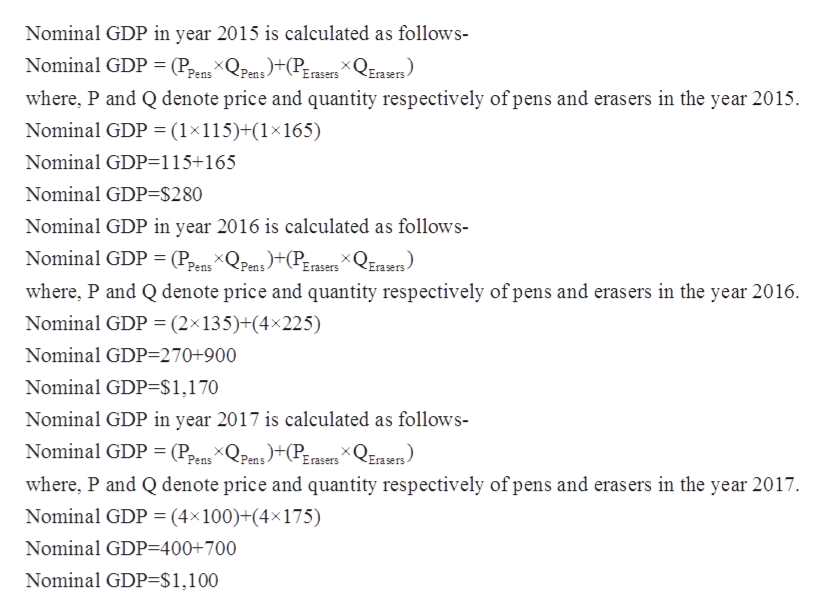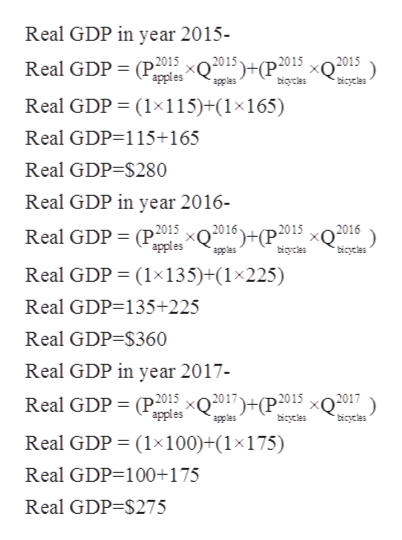# Consider a simple economy that produces two goods: pens and erasers. The following table shows the prices and quantities of the goods over a three-year period.YearPensErasersPriceQuantityPriceQuantity(Dollars per pen)(Number of pens)(Dollars per eraser)(Number of erasers)201511151165201621354225201741004175                     From 2016 to 2017, nominal GDP    , and real GDP    . The inflation rate in 2017 was    . Why is real GDP a more accurate measure of an economy's production than nominal GDP?Real GDP does not include the value of intermediate goods and services, but nominal GDP does. Real GDP includes the value of exports, but nominal GDP does not. Real GDP is not influenced by price changes, but nominal GDP is.

Question
660 views
Consider a simple economy that produces two goods: pens and erasers. The following table shows the prices and quantities of the goods over a three-year period.
Year
Pens
Erasers
Price
Quantity
Price
Quantity
(Dollars per pen)
(Number of pens)
(Dollars per eraser)
(Number of erasers)
2015 1 115 1 165
2016 2 135 4 225
2017 4 100 4 175

From 2016 to 2017, nominal GDP    , and real GDP    .

The inflation rate in 2017 was    .

Why is real GDP a more accurate measure of an economy's production than nominal GDP?
Real GDP does not include the value of intermediate goods and services, but nominal GDP does.

Real GDP includes the value of exports, but nominal GDP does not.

Real GDP is not influenced by price changes, but nominal GDP is.

check_circle

Step 1

Nominal GDP is the market value of all final goods and services produced by a nation at the current market prices. It does not take inflation in account and can be calculated by adding  revenue generated from all the goods and services produced in an year. Nominal GDP for all the three years is calculated as follows-help_outlineImage TranscriptioncloseNominal GDP in year 2015 is calculated as follows- Nominal GDP (Pens QPens+(Pasers x QErasers) where, P and Q denote price and quantity respectively of pens and erasers in the year 2015 Nominal GDP (1x115)+(1x165) Nominal GDP-115+165 Nominal GDP-\$280 Nominal GDP in year 2016 is calculated as follows- Nominal GDP (Pens XQPens+Pasers x QErasers) where, P and Q denote price and quantity respectively of pens and erasers in the year 2016 Nominal GDP = (2x135)+(4x225) Nominal GDP=270+900 Nominal GDP=\$1,170 Nominal GDP in year 2017 is calculated as follows- Nominal GDP (Pens XQPens+Prasers x QErasers) where, P and Q denote price and quantity respectively of pens and erasers in the year 2017 Nominal GDP (4x100)+(4x175) Nominal GDP=400+700 Nominal GDP=\$1,100 fullscreen
Step 2

From 2016 to 2017, the nominal GDP decreased from \$1,170 to \$1,100.

Step 3

Real GDP is market value of all goods and services evaluated at base year prices. Thus it is also called inflation adjusted GD...help_outlineImage TranscriptioncloseReal GDP in year 2015- O 2015 ) Real GDP= (P2015 x02015)+(P2015 apples apples bicles bicycles Real GDP (1x115)+(1x165) Real GDP-115+165 Real GDP-\$280 Real GDP in year 2016- Real GDP (P2015 apples

### Want to see the full answer?

See Solution

#### Want to see this answer and more?

Solutions are written by subject experts who are available 24/7. Questions are typically answered within 1 hour.*

See Solution
*Response times may vary by subject and question.
Tagged in